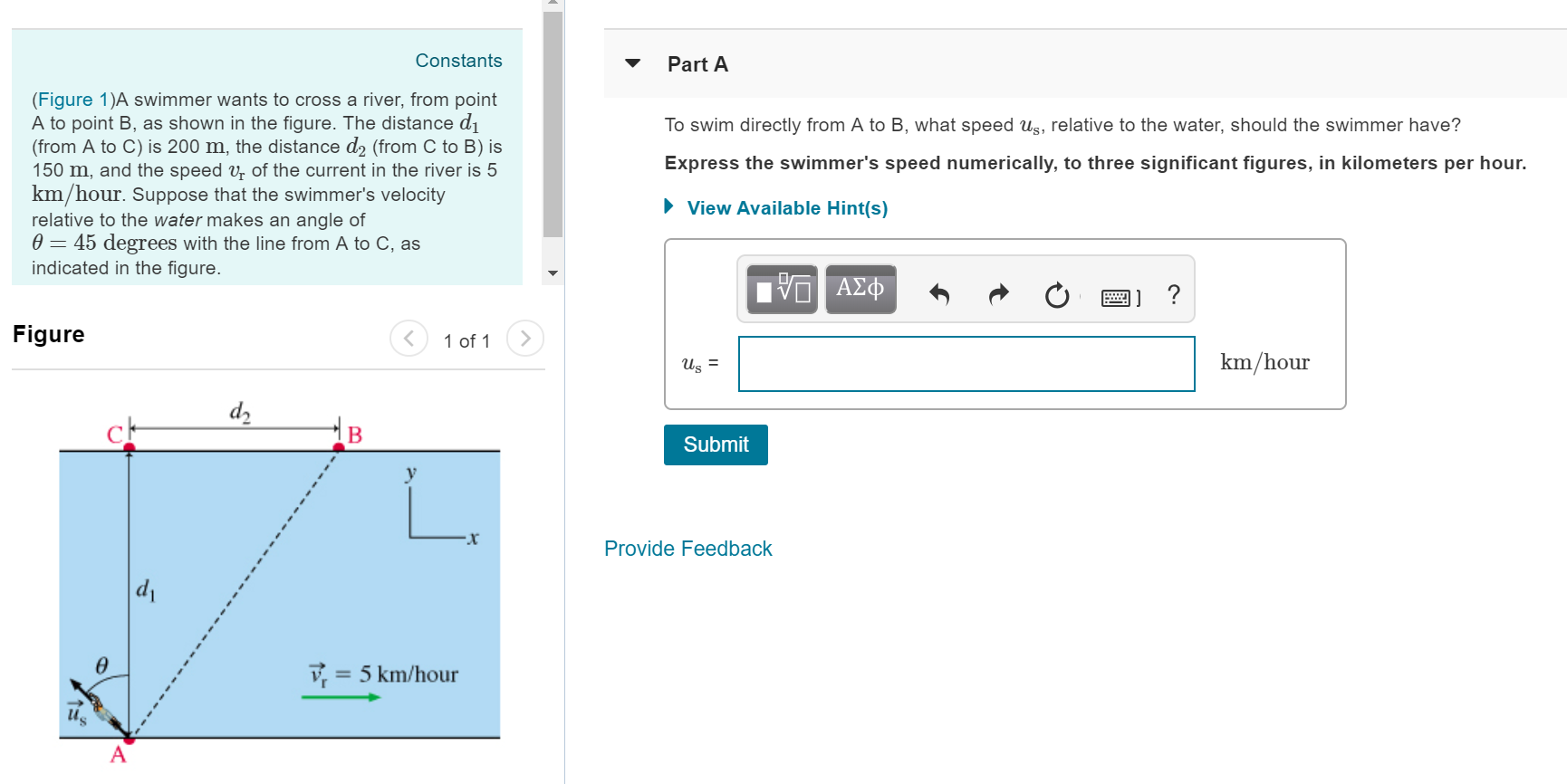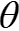# ConstantsPart A(Figure 1)A swimmer wants to cross a river, from pointA to point B, as shown in the figure. The distance difrom A to C) is 200 m, the distance d2 (from C to B) is150 m, and the speed vr of the current in the river is 5km/hour. Suppose that the swimmer's velocityrelative to the water makes an angle ofθ-45 degrees with the line from A to C, asindicated in the figureTo swim directly from A to B, what speed us, relative to the water, should the swimmer have?Express the swimmer's speed numerically, to three significant figures, in kilometers per hour.View Available Hint(s)Figure1 of 1>km/hourSubmitProvide Feedbackv,5 km/hour

Question
166 viewshelp_outlineImage TranscriptioncloseConstants Part A (Figure 1)A swimmer wants to cross a river, from point A to point B, as shown in the figure. The distance di from A to C) is 200 m, the distance d2 (from C to B) is 150 m, and the speed vr of the current in the river is 5 km/hour. Suppose that the swimmer's velocity relative to the water makes an angle of θ-45 degrees with the line from A to C, as indicated in the figure To swim directly from A to B, what speed us, relative to the water, should the swimmer have? Express the swimmer's speed numerically, to three significant figures, in kilometers per hour. View Available Hint(s) Figure 1 of 1> km/hour Submit Provide Feedback v, 5 km/hour fullscreen
check_circle

Step 1

Given,

Distance in y-direction, d1 = 0.2 km

Distance in x-direction, d2 = 0.15 km

Velocity of river, vr = 5 km/hr

Angle of swimmer velocity with the vertical direction,= 45o

Step 2

Displacement by swimmer.

Step 3

Relative velocity of swimmer w.r.t river = us

Velocity o...

### Want to see the full answer?

See Solution

#### Want to see this answer and more?

Solutions are written by subject experts who are available 24/7. Questions are typically answered within 1 hour.*

See Solution
*Response times may vary by subject and question.
Tagged in

### Vectors and Scalars